Example Questions

Example Question #31 : Exponential Operations

Simplify: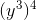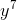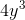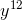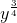Explanation:

When an exponent is raised to an exponent, multiply the two together to yield a new exponent, and attach that exponent to the original base.

In this case, multiplyandto yield, and your answer is.

Example Question #32 : Exponential Operations

Solve: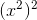when.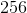Explanation:

When an exponent is raised to another exponent, you may multiply them together to find the exponent of the answer. Attach that exponent to the original base, and that is the solution.

In this particular problem, multiplyby, which yieldsas the final exponent. This makes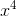.

Now, substitutefor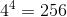.

Example Question #33 : Exponential Operations

What is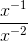?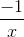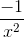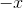Explanation:

Negative exponents are reciprocals. Do not change the sign to negative.

The variables with negative exponents can be written as their reciprocals.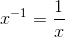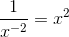,

Multiplying these new forms together gives,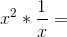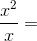From here, simplify by factoring out a common factor from the numerator and denominator.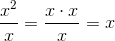Example Question #34 : Exponential Operations

Simplify the following: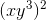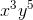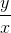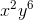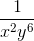Explanation:

To solve, simply distribute the outer exponent. When you have an exponent to an exponent, you multiply their values. Thus,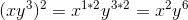Example Question #35 : Exponential Operations

Which of the following is a value of x that satisfies1

2

0

4

3

2

Explanation:

This question incorporates properties of exponents. The best way to solve this problem is to establish the same base for all of the terms.Now use the properties of exponents to simplify the left side of the equation. When exponential terms with the same base are multiplied, the exponents are added.Now, with the left side simplified, set that equal to.Since each side has one term with the same base, simply set the exponents equal to each other and solve for x.Example Question #1 : How To Add Exponents

For all x, 2x2 times 12x3 equals...

14x5

24x6

14x6

0

24x5

24x5

Explanation:

You multiply the integers, then add the exponents on the x's, giving you 24x5.

Example Question #2 : How To Add Exponents

Multiply: 2x² * 3x

6x²

6x3

5x

5x3

2x3

6x3

Explanation:

When multiplying exponents you smiply add the exponents.

For 2x² times 3x, 2 times 3 is 6, and 2 + 1 is 3, so 2x² times 3x = 6x3

Example Question #3 : How To Add Exponents

What is 23 + 22 ?

20

32

12

64

12

Explanation:

Using the rules of exponents, 23 + 22 = 8 + 4 = 12

Example Question #4 : How To Add Exponents

Solve forwhere: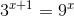5

9

3

1

2

1

Explanation:

The only value of x where the two equations equal each other is 1. All you have to do is substitute the answer choices in for x.

Example Question #5 : How To Add Exponents

A particle travels 9 x 107 meters per second in a straight line for 12 x 10-6 seconds. How many meters has it traveled?

1.08 x 105

1.08 x 103

1.08 x 10

1.08